Courses

# Phase Controlled Rectifiers - 1

## 10 Questions MCQ Test GATE Electrical Engineering (EE) 2022 Mock Test Series | Phase Controlled Rectifiers - 1

Description
This mock test of Phase Controlled Rectifiers - 1 for Electrical Engineering (EE) helps you for every Electrical Engineering (EE) entrance exam. This contains 10 Multiple Choice Questions for Electrical Engineering (EE) Phase Controlled Rectifiers - 1 (mcq) to study with solutions a complete question bank. The solved questions answers in this Phase Controlled Rectifiers - 1 quiz give you a good mix of easy questions and tough questions. Electrical Engineering (EE) students definitely take this Phase Controlled Rectifiers - 1 exercise for a better result in the exam. You can find other Phase Controlled Rectifiers - 1 extra questions, long questions & short questions for Electrical Engineering (EE) on EduRev as well by searching above.
QUESTION: 1

### In an SCR half wave rectifier, the forward breakdown voltage of SCR is 110 V for a gate current of 1 mA. If a 50 Hz sinusoidal voltage of 220 V peak is applied, the time during which SCR remains OFF would be equal to (assume load resistance is 100 Ω and the holding current to be zero)

Solution: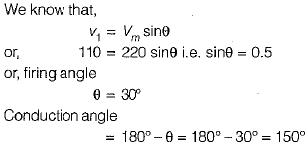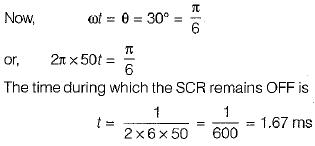QUESTION: 2

### When an SCR full wave rectifier is connected across a sinusoidal voltage of 400 sin 314t, the RMS value of the current flowing through the device is 20 A. The power rating of the SCR is

Solution:

As the supply voltage is 400 sin 314t
Vm = 400 V,
Peak invesre voltage (PIV)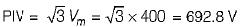RMS value of current = 20 A
Average value of current,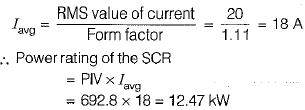QUESTION: 3

### Assertion (A): Single phase half-wave converter introduces a dc component into the supply line. Reason (R) : The supply current taken from the source is unidirectional and is in the form of dc pulses

Solution:
QUESTION: 4

Assertion (A) : By using freewheeling diode, load performance becomes better.
Reason ( R ) : Freewheeling diode prevents the load voltage from becoming negative.

Solution:

By using freewheeling diode, load performance becomes better because load current waveform is improved.
Whenever load voltage tends to go negative, freewheeling comes into play as a result of which ioaa current is transferred from main thyristor to freewheeling diode, allowing the thyristor to regain its forward blocking capability.
Hence, both assertion and reason are true but reason is not the correct explanation of assertion.

QUESTION: 5

The advantage of using a freewheeling diode with bridge type ac to dc converter is

Solution:

Power delivered to the load, for the same firing angle is more when freewheeling diode is used As volt-ampere input is almost the same witt and without a freewheeling diode, therefore the input power factor with use of freewheeling diode is improved.

QUESTION: 6

A single-phase full converter is connected across 230 V ac. Its average output voltage when firing angle is 30° is approximately equal to

Solution:

The average output voltage for a single-phase full converter is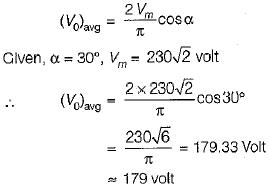QUESTION: 7

SCRs with peak forward rating of 1 kV and average on-state current rating of 40 A is used in single-phase mid-point converter. If the factor of safety is 2.5, then the power that this converter can handle is

Solution:

Maximum voltage across SCR in single-phase mid-point converter is 2Vm. Therefore, this converter can be design for a maximum voltage of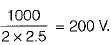∴ Maximum average power that the mid-point converter can handle is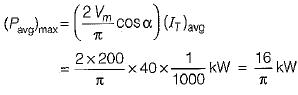QUESTION: 8

The main advantages of the dual converter with circulating current operation is/are
1. the efficiency and power factor are more.
2. it has faster operation than dual converter without circulating current.
3. the thyristors are rated for lower current.

Choose the correct code from the following

Solution:

Circulating current gives rise to more losses in the converters, hence the efficiency and power factor are low. Thus, statement-1 is false.
In a non-circulating current type dual converter, the normal delay period of 10 to 20 ms is required while no delay period is required in circulating current type dual converter. Therefore, circulating current type dual converter is faster than non-circulating type dual converter. Thus, statement-2 is true.
As the dual converter with circulating current has to handle load current as well as circulating currents, therefore the thyristors are rated for higher currents. Hence statement-3 is false.

QUESTION: 9

The average value of voltage of a single-phase ac voltage converter with resistive load is (α is the firing angle).

Solution:
QUESTION: 10

A four quadrant operation requires

Solution:

In case “four quadrant operation" is required without any mechanical changeover switch, two full converters can be connected back to back to the load circuit. Such an arrangement using two converters in antiparailel and connected to the same dc load is called a “dual converter."